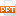# Chapter 5 Inverse Functions - Section 5.7: Hyperbolic functions

Likewise, if t is any real number, then the point P(cosh t, sinh t) lies on the right branch of the hyperbola x2 – y2 = 1 because cosh2 t – sin2 t = 1 and cosh t ≥ 1. This time, t does not represent the measure of an angle.21 trang | Chia sẻ: vutrong32 | Ngày: 19/10/2018 | Lượt xem: 285 | Lượt tải: 0
Bạn đang xem trước 20 trang tài liệu Chapter 5 Inverse Functions - Section 5.7: Hyperbolic functions, để xem tài liệu hoàn chỉnh bạn click vào nút DOWNLOAD ở trên
SECTION 5.7HYPERBOLIC FUNCTIONSP*5.7INVERSE FUNCTIONSCertain combinations of the exponential functions ex and e–x arise so frequently in mathematics and its applications that they deserve to be given special names. In many ways they are analogous to the trigonometric functions, and they have the same relationship to the hyperbola that the trigonometric functions have to the circle. For this reason they are collectively called hyperbolic functions and individually called hyperbolic sine, hyperbolic cosine, and so on. P*5.7DEFINITION OF THE HYPERBOLIC FUNCTIONSP*5.7HYPERBOLIC FUNCTIONSThe graphs of hyperbolic sine and cosine can be sketched using graphical addition, as in Figures 1 and 2.P*5.7HYPERBOLIC FUNCTIONSNote that sinh has domain and range , whereas cosh has domain and range .P*5.7HYPERBOLIC FUNCTIONSThe graph of tanh is shown in Figure 3. It has the horizontal asymptotes y = ±1.P*5.7APPLICATIONSApplications of hyperbolic functions to science and engineering occur whenever an entity such as light, velocity, electricity, or radioactivity is gradually absorbed or extinguished, for the decay can be represented by hyperbolic functions.P*5.7APPLICATIONSThe most famous application is the use of hyperbolic cosine to describe the shape of a hanging wire.P*5.7APPLICATIONSIt can be proved that, if a heavy flexible cable is suspended between two points at the same height, it takes the shape of a curve with equation y = c + a cosh(x/a) called a catenary.See Figure 4.The Latin word catena means ‘‘chain.’’P*5.7HYPERBOLIC IDENTITIESThe hyperbolic functions satisfy a number of identities that are similar to well-known trigonometric identities.P*5.7HYPERBOLIC IDENTITIESP*5.7Example 1Prove (a) cosh2x – sinh2x = 1 (b) 1 – tanh2x = sech2xSOLUTION (a)P*5.7Example 1(b) SOLUTIONWe start with the identity proved in (a) cosh2x – sinh2x = 1If we divide both sides by cosh2x, we get:P*5.7HYPERBOLIC FUNCTIONSThe identity proved in Example 1(a) gives a clue to the reason for the name ‘hyperbolic’ functions, as follows:P*5.7HYPERBOLIC FUNCTIONSIf t is any real number, then the point P (cos t, sin t) lies on the unit circle x2 + y2 = 1 because cos2 t + sin2 t = 1. In fact, t can be interpreted as the radian measure of in Figure 5.For this reason, the trigonometric functions are sometimes called circular functions.P*5.7HYPERBOLIC FUNCTIONSLikewise, if t is any real number, then the point P(cosh t, sinh t) lies on the right branch of the hyperbola x2 – y2 = 1 because cosh2 t – sin2 t = 1 and cosh t ≥ 1.This time, t does not represent the measure of an angle. P*5.7HYPERBOLIC FUNCTIONSHowever, it turns out that t represents twice the area of the shaded hyperbolic sector in Figure 6.This is just as in the trigonometric case t represents twice the area of the shaded circular sector in Figure 5.P*5.7DERIVATIVES OF HYPERBOLIC FUNCTIONSThe derivatives of the hyperbolic functions are easily computed. For example,P*5.7DERIVATIVES OF HYPERBOLIC FUNCTIONSWe list the differentiation formulas for the hyperbolic functions as Table 1. P*5.7DERIVATIVES OF HYPERBOLIC FUNCTIONSNote the analogy with the differentiation formulas for trigonometric functions.However, beware that the signs are different in some cases.P*5.7Example 2Any of these differentiation rules can be combined with the Chain Rule. SOLUTIONFor instance,

Các file đính kèm theo tài liệu này:

•hyperbolic_functions_2602.ppt
Tài liệu liên quan